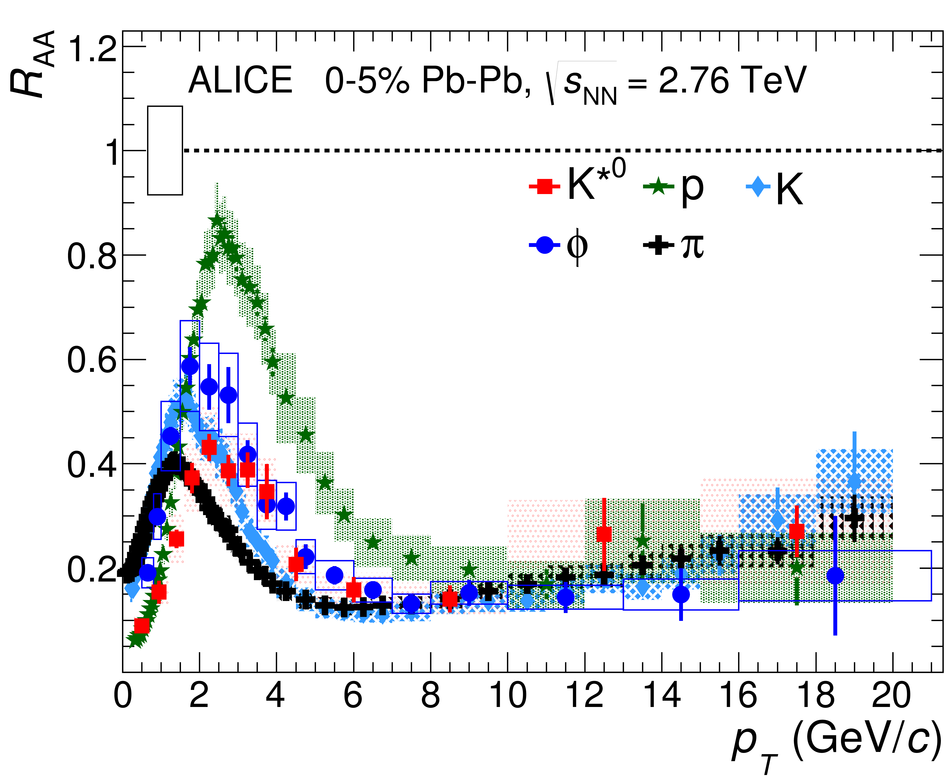# K$^{*}(892)^{0}$ and $φ(1020)$ meson production at high transverse momentum in pp and Pb-Pb collisions at $\sqrt{s_\mathrm{NN}}$ = 2.76 TeV

The production of K$^{*}(892)^{0}$ and $\phi(1020)$ mesons in proton-proton (pp) and lead-lead (Pb-Pb) collisions at $\sqrt{s_\mathrm{NN}} =$ 2.76 TeV has been analyzed using a high luminosity data sample accumulated in 2011 with ALICE detector at the Large Hadron Collider (LHC). Transverse momentum ($p_{\mathrm{T}}$) spectra have been measured for K$^{*}(892)^{0}$ and $\phi(1020)$ mesons via their hadronic decay channels for $p_{\mathrm{T}}$ up to 20 GeV/$c$. The measurements in pp collisions have been compared to model calculations and used to determine the nuclear modification factor and particle ratios. The K$^{*}(892)^{0}$/K ratio exhibits significant reduction from pp to central Pb-Pb collisions, consistent with the suppression of the K$^{*}(892)^{0}$ yield at low $p_{\mathrm{T}}$ due to rescattering of its decay products in the hadronic phase. In central Pb-Pb collisions the $p_{\mathrm{T}}$ dependent $\phi(1020)/\pi$ and K$^{*}(892)^{0}$/$\pi$ ratios show an enhancement over pp collisions for $p_{\mathrm{T}}$ $\sim$3 GeV/$c$, consistent with previous observations of strong radial flow. At high $p_{\mathrm{T}}$, particle ratios in Pb-Pb collisions are similar to those measured in pp collisions. In central Pb-Pb collisions, the production of K$^{*}(892)^{0}$ and $\phi(1020)$ mesons is suppressed for $p_{\mathrm{T}}> 8$ GeV/$c$. This suppression is similar to that of charged pions, kaons and protons, indicating that the suppression does not depend on particle mass or flavor in the light quark sector.

Figures

## Figure 1

 Invariant-mass distributions of $\pi$K pairs for pp and the 0-5$\%$ most central Pb-Pb collisions at $\sqrt{s_{\rm{NN}}}$ = 2.76 TeV for the momentum ranges 0.6 < $p_{\rm{T}}$ < 0.9 GeV/$c$ (upper panel) and 10 < $p_{\rm{T}}$ < 15 GeV/$c$ (lower panel), respectively. Panels (a) and (c) show the unlike charge $\pi$K invariant-mass distribution from the same event and normalized mixed event background. Panels (b) and (d) report the invariant-mass distribution after subtraction of the combinatorial background for K$^{*0}$. The statistical uncertainties are shown by bars. The solid curves represent fits to the distributions and the red dashed curves are the components of those fits that describe the residual background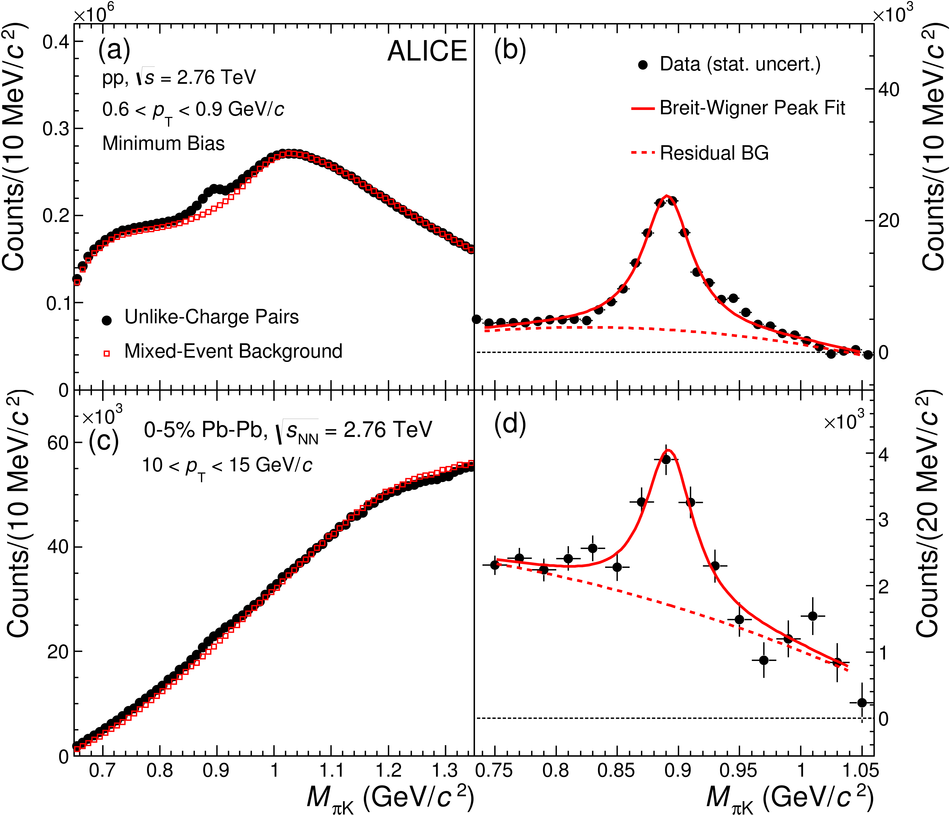## Figure 2

 Invariant-mass distributions of KK pairs for pp and the 0-5$\%$ most central Pb-Pb collisions at $\sqrt{s_{\mathrm{NN}}}$ = 2.76 TeV for the momentum ranges 0.5 < $p_{\mathrm{T}}$ < 0.8 GeV/$c$ (upper panel) and 10 < $p_{\mathrm{T}}$ < 13 GeV/$c$ (lower panel), respectively. In panels (a) and (c) the unlike charge KK invariant-mass distribution from the same event and normalized mixed event background are shown. In panels (b) and (d) the invariant-mass distribution after subtraction of the combinatorial background for $\phi$ is shown. The statistical uncertainties are shown by bars. The solid curves are the fits to the distributions and the red dashed curves are the components of those fits that describe the residual background.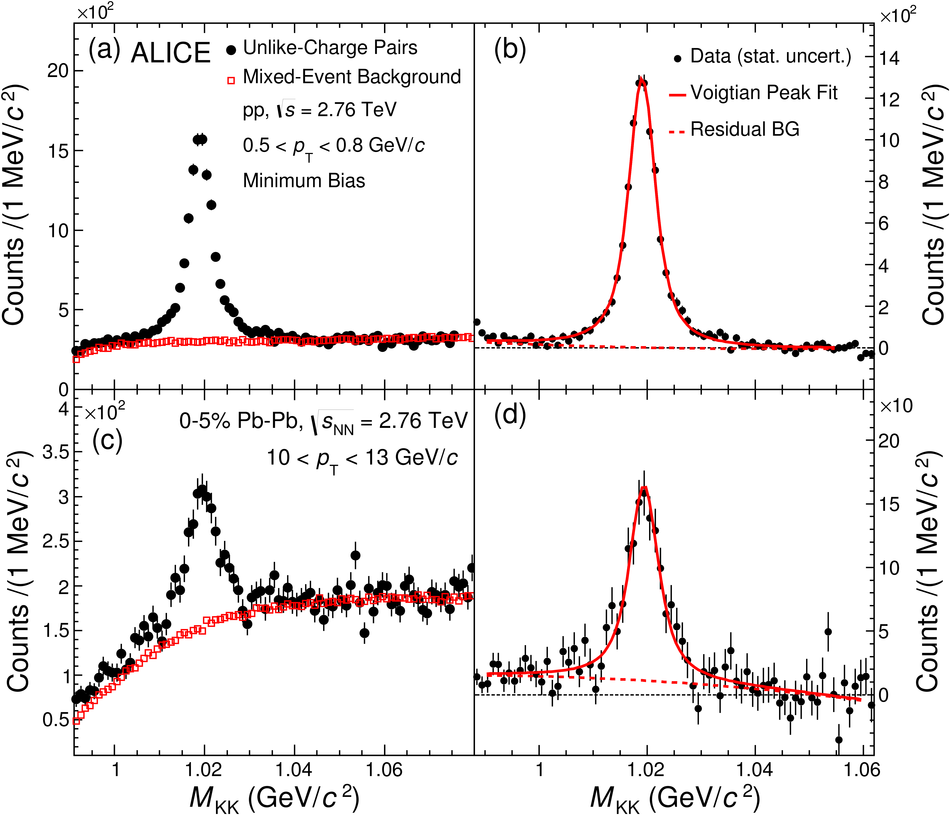## Figure 3

 The acceptance and efficiency ($A \times$ $\epsilon_{\mathrm{rec}}$) correction as a function of $p_{\mathrm{T}}$ for K$^{*0}$ (red marker) and $\phi$ (blue marker) mesons in pp (left panel) and 0-5$\%$ centrality in Pb-Pb (right panel) collisions at $\sqrt{s_\mathrm{NN}}$ = 2.76 TeV.## Figure 4

 Invariant yields for (a) K$^{*0}$ and (b) $\phi$ mesons normalized to the number of inelastic pp collisions at $\sqrt{s}$ = 2.76 TeV. Invariant yield is calculated by taking the value of $p_{\mathrm{T}}$ at the corresponding bin center. The statistical uncertainties on the data are shown by bars and the systematic uncertainties by boxes. The results are compared with model calculations from PYTHIA 8.14 , PHOJET [34,35], PYTHIA D6T , PYTHIA ATLAS-CSC  and PYTHIA PERUGIA  as shown by different dashed lines. The lower panel for both K$^{*0}$ and $\phi$ shows the model to data ratio.## Figure 5

 Invariant yields of (a) K$^{*0}$ and (b) $\phi$ mesons in various centrality classes in Pb-Pb collisions at $\sqrt{s_\mathrm{NN}}$ = 2.76 TeV Invariant yield is calculated by taking the value of $p_{\mathrm{T}}$ at the corresponding bin center. The statistical and systematic uncertainties are shown as bars and boxes, respectively. The normalization uncertainty is not shown here, but is given in Tab. 3.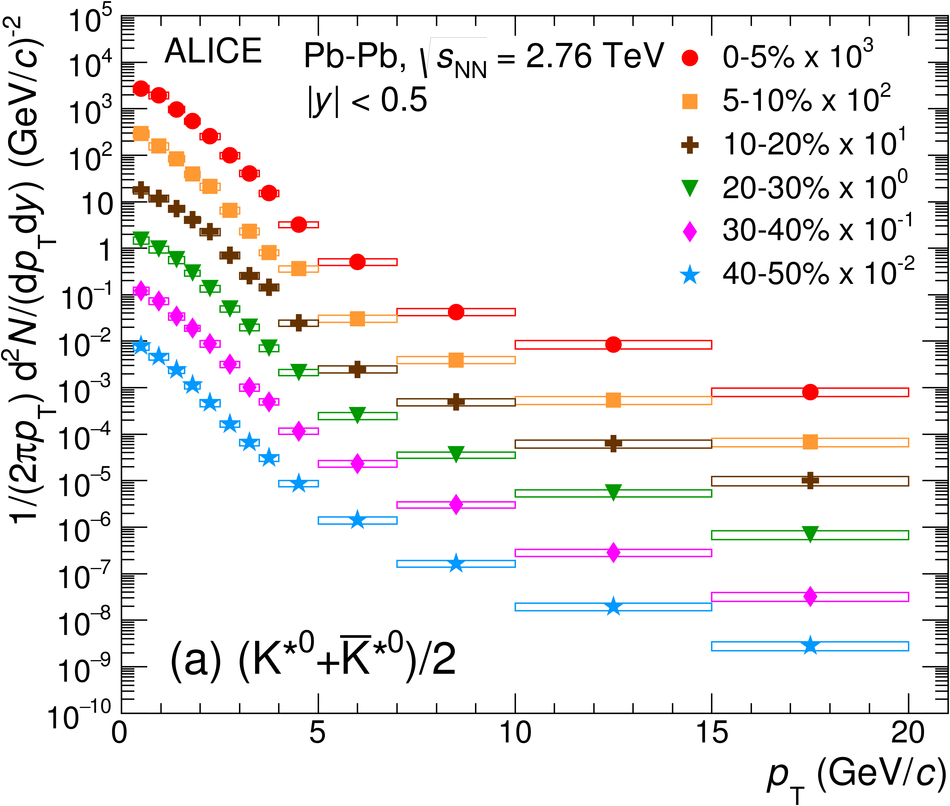## Figure 6

 K$^{*0}$/K$^{-}$ and $\phi$/K$^{-}$ ratios as a function of $\langle\mathrm{d}N_{\mathrm{ch}}/\mathrm{d}\eta\rangle^{1/3}$ measured at mid-rapidity  in pp collisions at $\sqrt{s}$ = 2.76 TeV and 7 TeV , and Pb-Pb collisions at $\sqrt{s_\mathrm{NN}}$ = 2.76 TeV. For Pb--Pb collisions, the $\phi$/K$^{-}$ values are exclusively from ; the previously published K$^{*0}$/K$^{-}$ measurements are compared to new measurements in finer centrality classes. Bars represent the statistical uncertainties, empty boxes represent the total systematic uncertainties, and shaded boxes represent the systematic uncertainties that are uncorrelated between centrality classes. The expectations from a thermal model calculation with chemical freeze-out temperature of 156 MeV for the most central collisions  are shown. The EPOS3 calculation of the K$^{*0}$/K and $\phi$/K ratios are also shown as a violet band for different centrality intervals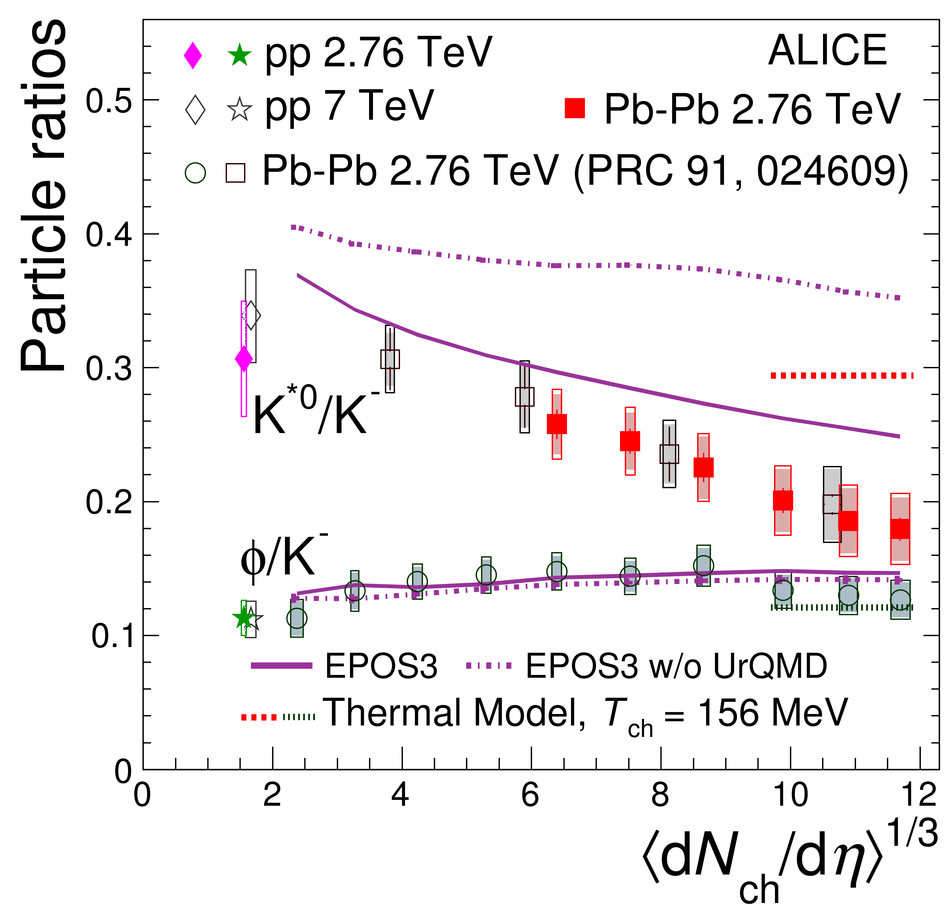## Figure 7

 Ratios of particle yields K$^{*0}$/K and $\phi/$K in panel (a) and K$^{*0}/\pi$ and $\phi/\pi$ in panel (b) as a function of $p_{\mathrm{T}}$ in central Pb--Pb and pp collisions at $\sqrt{s_\mathrm{NN}}$ = 2.76 TeV are shown. Here, (K$^{*0}$+$\overline{\mathrm{K}}^{*0}$), ($\mathrm{K}^{+}$+$\mathrm{K}^{-}$) and ($\pi^{+}$+$\pi^{-}$) are denoted as K$^{*0}$, K, $\pi$, respectively. The statistical and systematic uncertainties are shown as bars and caps respectively.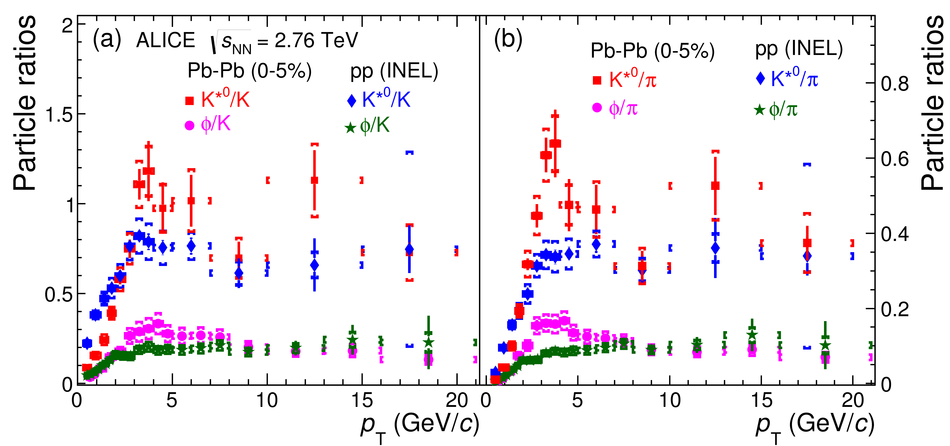## Figure 8

 Ratios of particle yields p/K$^{*0}$ in panel (a) and p/$\phi$ in panel (b) as a function of $p_{\mathrm{T}}$ in central and peripheral Pb--Pb collisions and pp collisions at $\sqrt{s_\mathrm{NN}}$ = 2.76 TeV. The p/$\phi$ ratio for $p_{\mathrm{T}}$ < 4 GeV/$c$ is from . The statistical and systematic uncertainties are shown as bars and boxes respectively.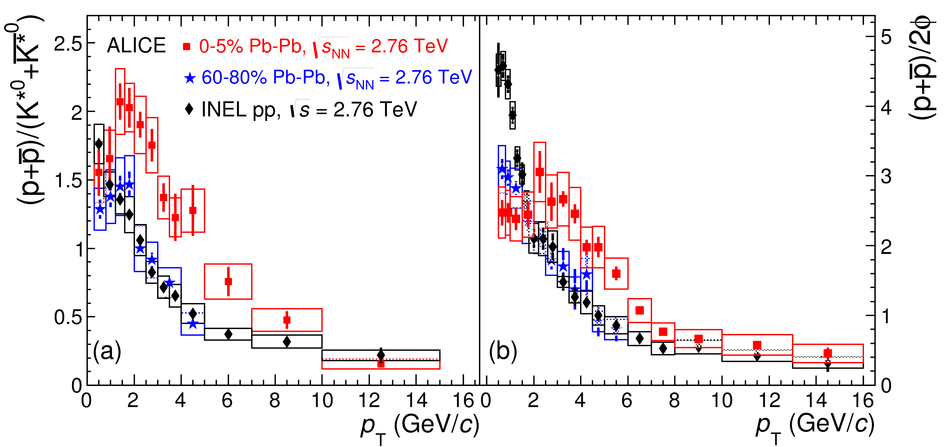## Figure 9

 The nuclear modification factor, $R_\mathrm{AA}$, as a function of $p_{\mathrm{T}}$ for K$^{*0}$ and $\phi$ mesons in Pb-Pb collisions for different centrality classes. The results are compared with the $R_\mathrm{AA}$ of charged hadrons measured by ALICE . The statistical and systematic uncertainties are shown as bars and boxes, respectively. The boxes around unity indicate the uncertainty on the normalization of $R_\mathrm{AA}$, including the uncertainty on the nuclear overlap function $\langle T_{\mathrm{AA}} \rangle$ and the normalization uncertainty given in Table 3.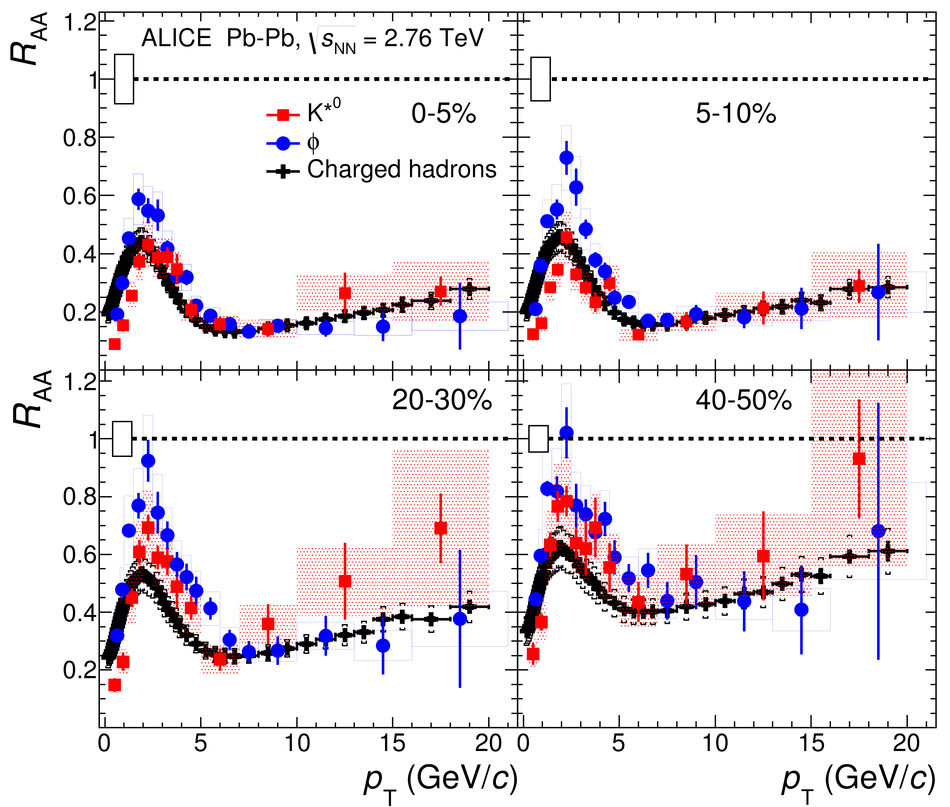## Figure 10

 The $R_\mathrm{AA}$ for K$^{*0}$ and $\phi$ mesons as a function of $p_{\mathrm{T}}$ in 0-5$\%$ Pb-Pb collisions. The results are compared with the $R_\mathrm{AA}$ of $\pi$, K and p  The statistical and systematic uncertainties are shown as bars and boxes, respectively. The boxes around unity indicate the uncertainty on the normalization of $R_\mathrm{AA}$, including the uncertainty on the nuclear overlap function $\langle T_{\mathrm{AA}} \rangle$ and the normalization uncertainty given in Table 3.